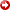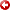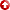[First Hit]MARSCHALL, R. (OFS), H.-J. ZOCH (RWE-DEA), C. HENKE (RWE-DEA), M. KRIEGER (TERRASYS), F. KOCKEL (BGR)

Abstract: Successful imaging below salt: technique and 2 case histories

Summary:

Areas with either volcanic basalt intrusions or intensive salt-tectonics require sophisticated methods in order to solve the sub-basalt or sub-salt imaging problem.To be specific, it is at the beginning not an imaging problem, but an illumination problem, which then manifests itself as an imaging problem due to the propagation effects of the wavefields involved.

While for basalt-covered areas inprincipletwo approaches exist to solve the problem, i.e. Wide-Angle acquisition and/or consideration of local S-mode-conversions in the P-ray paths, for salt-related problems the geometry of the salt body represents the dominant quantity for the methods available: either prestack-depth migration based on the correct macro model and/or the undershooting method (here again superlong offsets are involved in order to avoid those parts in the overburden where the large velocity contrasts sediment/salt occur). In addition often local VSP- and MSP (moving source profiles)-surveys may be used as well to define/constrain locally the macro model. Here in case of overhangs also the so-called "turning-wave"- migration technique may be used.

Global constraints are obtained in conjunction with additional 3D-gravity surveys (if acquired).

Introduction:

Two examples for salt-related problems will be discussed, i.e.
- an oil field, accumulated in a typical Jurassic trough confined by long North-South trending salt walls of different age including a local overhang-problem
- a gas field (Rotliegendes), located below an extensive Zechstein salt structure. This case history is of particular interest, since here the first 2-D and 3-D-Undershooting surveys have been carried out.

For both cases gravity data were used as well in order to constrain the macro model needed for proper imaging.

As the examples will show, 3-D Seismics in conjunction with special techniques like Undershooting, MSP-surveys and Gravity data guide the way to correct solutions.

Methodology:

The results obtained by seismic data strongly depend on three basic factors:
- Selection of appropriate 3D-acquisition geometry
- Selection of appropriate processing strategy and finally
- appropriate interpretation (structurally as well as sequence-stratigraphically!). While the structural interpretation results in the proper "structural model" (=macro model), the sequence-stratigraphic interpretation is expected to result in the proper "litho-model" of the reservoir. Quality criteria to be used here include balancing techniques in conjunction with palinspastic restoration for the structural model, and the so-called "Verification loop" (Fischer et al.,1994) for the Lithomodel.

- Firstly the 3D-acquisition geometry has to be designed carefully following certain well-defined rules (Marschall, 1997).The 3 basic parameters here are a = size of acquisition-bin (surface), which should be a square (dimension: a times a). Smax= maximum offset: this parameter defines the upper limit of offset with respect to a given target. C3D = nominal 3D-coverage (which is factored into inline- (Cx) and crossline (Cy) coverage).While for land-acquisition schemes the normal case is that Cx = X/2, in the marine case the de fault value (unfortunately) for crossline coverage still is Cy = 1 (i.e. singlefold crossline!). This is due to the fact that land-geometries inprincipleare orthogonal geometries, while marine geometries are parallel geometries. For the marine case (due to this single-crossline-fold-property of the data) a considerable DMO-problem is created, which easily could be avoided by increasing the coverage: for the marine case the reference is a data-set with crossline-fold being equal to the actual number of streamers used. However, so in either case there exists an absolute reference relative to which the actual 3D-geometry has to be evaluated with respect to its properties in terms of Signal-to-Noise (S/N)-ratio.

- Based on these 3 parameters then the so-called "homogeneous scheme" (shots only between the central two receiver lines) is established.The properties of this scheme completely define its capabilities in terms of the final S/N-ratio to be expected. The homogeneous scheme is a subset of the corresponding well defined full-fold roll-along circle scheme (= absolute reference for S/N).This circle-scheme by definition exists for both, i.e. marine- as well as land-3D-data.The given value of C3D has to be factored into inline- and cross line coverage, i.e. Cx and Cy respectively.The actual value of Cx then immediately gives the number of receiver lines (X) to be used: X = number of receiver lines (with N channels each), resulting in X times N active channels per 3D experiment (=3D-shot), and we have: X = 2.Cx. Repeating K = X-1 receiver lines in crossline roll-along results in the homogeneous scheme: this homogeneous scheme constitutes the essential information to be evaluated in order to be able to design the desired optimum (with reference to certain constraints as there are e.g. groundroll, multiples etc) 3D-acquisition geometry.

Next step is to determine the number of different CMP-configurations resulting from the 3D-scheme.

This quantity is denoted by BSC and is obtained from the periodicity of the 3D-scheme in x- and y-direction, px and py respectively.This then gives: BSC = px.py/2.

The size of BSC is crucial, because exactly BSC different CMP-configurations result from the 3D-scheme, which are to be repeated all over the 3D-survey area, and which therefore determine the actual overall S/N-ratio in terms of multiple-suppression, groundroll suppression etc.

Having discussed briefly the 3D-design, we switch over now to processing of the resulting 3D-data.

To begin with we define that quantity which should be the final outcome of the processing exercise:
it is a Zero-Offset-volume, properly migrated and ready for interpretation in both, i.e. time-and depth-domain, having a uniform S/N-ratio.

This objective is reached by applying the following strategy:
We subdivide processing into three distinct phases (=domains), i.e. time-domain, poststack-depth-domain and prestack-depth-domain. In addition we define the essential criterion, which drives these three domains: it is the Zero Offset-condition. This condition is of utmost importance: if it is valid at stack-stage, then the corresponding 3D-volume represents the perfect input to the subsequent migration process, be it in time-domain or poststack depth-domain (we tacitly assume here that a reliable wave-equation based algorithm is to be used!).

A more detailed discussion follows:

A) TIME DOMAIN: this part constitutes the conventional processing sequence up to DMO-stack and subsequent time domain migration.The basic function of the DMO-process (Dip Move Out) is the mapping of the seismic data towards zero offset. This is achieved by a partial prestack migration- process, which represents an approximation and therefore possibly results in local errors in the result, which have to be investigated. The easiest way to achieve this check is to simply decimate the given seismic volume, i.e. a subvolume is established by cancelling all offsets beyond a user-defined threshold (as an example this could be an offset of, say, 500 meters).The resulting subset often is called a Near Trace (NT)-section. Comparing then  after migration the nominal full-fold result with the NT-migration result reveals immediately if the two results are equivalent in terms of structural information (in terms of S/N-ratio the NT-result is inferior by definition due to its lower coverage). If there are "no" differences between these two results then the conclusion here is that the DMO- stack constitutes the "perfect" Zero Offset-Volume, and its time migration is a valid result. If, however, there are differences, then the only way to improve the result is the application of phase three, i.e. prestack depth migration in order to avoid the DMO-approximation.The interpreter has to make this decision. So the essential condition here is the validity (or violation) of the ZO-condition. Considering the case of valid ZO-condition first, one has to recall that time migration by definition assumes the presence of a laterally smooth velocity model V(x,y,t). This assumption of course is incorrect and as the direct consequence the result of time migration shows local residual errors , becauseFermat'sprinciplelocally is violated. But this is not a problem (we assume here the validity of the ZO-condition), since the result has to be transformed to depth-domain anyway. Unfortunately here often a shortcut is taken: the time migration result is simply depth-stretched (vertical stretch) in order to obtain the result in depth-domain.This approximation, however, should be avoided for two reasons: - for the depth stretch usually a velocity field is used, which "has nothing to do" with the processing- velocity field. This means that an important piece of information is totally ignored: it is to be recalled that in acquisition we measure offsets and travel times (we look at the kinematic aspects here). The depth-domain transformation has to use a macro-model ( velocity-interfaces and interval-velocities), which is obtained by interpreting the time migration, i.e. now we "sample geology" by replacing the seismic volume by a set of interfaces (usually between 5 and 10). If now the velocity-distribution is known for each interval, then the time-to-depth-transformation can be carried out, but this procedure should aim to correct for the inherent still present residual positioning error of the time migration result.This objective is reached by the IMAGE RAY APPROACH: an image-ray migration is carried out for the discrete interfaces resulting from interpretation of the time-migration result. However, for this step the velocity-distribution is mandatory. Often so-called regional velocity functions of the type V(z) = Vo + k.z (or Faust-type functions etc...) are used for this step. Since a valid macro model should have an accuracy of equal to or better than 1% one should use in addition the measured traveltimes and offsets of the seismic data at hand in order to correct/ improve/verify the validity and accuracy of the macro model. The objective here is that the final macro model has to explain what we measure, namely offsets and travel times for the interfaces selected.This brings us to the second reason: - Data-consistent macro models (i.e. macro models which explain measured offsets and travel times) can be (and should be) constructed. Basically two approaches are available, i.e. the "point"- and the "continuous event"-approach. Since the point-approach uses discrete CMP-information, the event- approach is superior. Here one digitizes Constant-Offset-Sections (COS) in terms of the interfaces selected and carries out a raypath-based depth migration of these COS-data. The interface in depth domain is obtained by the envelope-section to all resulting pseudo-ellipses (locus of constant travel time).All envelopes (the actual number depends on the number of COS used) in case of a correct velocity field coincide. In case of wrong velocities the depth-difference (measured along the ZO-ray) is used to obtain the local update (=correction for the velocity field) (Marschall, 1991, Zoch et al. 1993, Brauner et al., 1991). Note that this is a two-step procedure, not an endless iteration loop! If available, of course gravity data can be used to a great advantage (Krieger et al., 1998).

B) POSTSTACK DEPTH DOMAIN: Having built the macro model along above guide lines, the DMO-stack (and if necessary the NT-section) is poststack depth migrated and displayed in depth domain as well as in time domain (which is achieved by using the given macro model), since here the "frequency-stretch" caused by the depth- domain is avoided. Note that phase A and B should be executed for ALL data! Finally we come back to the case that the ZO-condition locally does NOT hold: for this case one simply continues (after phases A and B) with phase C, i.e.

C) PRESTACK DEPTH DOMAIN:This phase avoids the DMO-process at all, and usually is done on a shot-gather basis.The stack of the individually prestack depth migrated shots then constitutes the final result (of course an optimum mute, which may be derived macro-model driven as well, should be applied in addition to the "normal" result, i.e. a stack without any mute!).

Above strategy was applied successfully to the famous Marmousi-data set as well (Marschall&Thiessen, 1991). Synthetic data-results (Kuiper&Kikkert, 1998) confirm it as well.

Finally the last step takes place, i.e. structural and seismo-stratigraphic interpretation of the final image, resulting in the final structural model as well as litho-model. Usually the litho-model is verified by forward modeling, migration and comparison to the existing seismic data.

Data examples:

Two data examples will be shown, i.e. Mittelplate (oilfield, Jurassic trough, saltdome flank related) (Zoch et al., 1998) and Verden (gasfield "below"a large saltdome) (Apel et al., 1998).

In both cases above outlined strategy was applied for 2D- as well as 3D-data in conjunction with gravity data, and selected sections demonstrating the merits of the approach will be shown.

The Mittelplate case represents an oil field within a mesozoic overburden (Jurassic trough) of the Permian salt at the flank of a salt structure which controlled the sedimentation process.

In Mittelplate-oilfield currently extended-reach-drilling is ongoing, and the results so far have confirmed the interpretation. In addition during the acquisition of the 3-D transition zone survey (which was only possible under severe environmental constraints!) a Moving-Source Survey (MSP) was acquired by placing a 3C-receiver into a horizontal well, and using the seismic energy source (=airgun) along three lines. By using the downgoing wavefield of this survey the steeply dipping reservoir could be located as well by using wavefront methods (Brauner et al., 1988).

The Verden case represents a gas deposit within Lower Permian sandstones beneath an Upper Permian saltstructure, which was compressed and deformed during late Cretaceous inversion.This case is used to demonstrate the application of above strategy to a 2-D seismic line crossing the saltdome, and results for the individual stages of processing will be given.

Summary:

By applying a well defined sequence of steps (from acquisition to processing and interpretation) excellent results in terms of structural and sequence-stratigraphic objectives are achieved. The essential ingredients here are reliable, data-consistent macro models in conjunction with additional gravity data and a well-defined processing- and interpretation strategy.

AAPG Search and Discovery Article #[email protected] International Conference and Exhibition, Birmingham, England